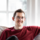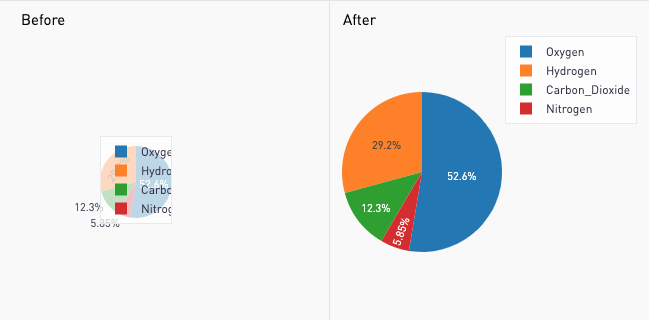# Remove Whitespace Margins from Plotly Charts

Plotly is wonderful, but sometimes you end up with a chart that looks like it's too small, or that it's not taking up all the space. Why does it do that?

In this post, you'll learn how to fix that:With Python, this is actually very easy. We just need to add layout specifications. The documentation for the layout settings can be found on Plotly's website here.

```layout = go.Layout(
margin=go.layout.Margin(
l=0, #left margin
r=0, #right margin
b=0, #bottom margin
t=0, #top margin
)
)

fig = dict(data=data, layout=layout)
```

Voila! There you go. The default values for the margins are 80 pixels on the left, right, and bottom and 100 pixels on the top. We don't need those! Note: A chart with axes that need to be labeled might need about 25 pixels on the bottom and left.

Before

```import plotly.plotly as py
import plotly.graph_objs as go

labels = ['Oxygen','Hydrogen','Carbon_Dioxide','Nitrogen']
values = [4500,2500,1053,500]

trace = go.Pie(labels=labels, values=values)

data = [trace]
fig = dict(data=data)

periscope.plotly(fig)```

After

```import plotly.plotly as py
import plotly.graph_objs as go

labels = ['Oxygen','Hydrogen','Carbon_Dioxide','Nitrogen']
values = [4500,2500,1053,500]

trace = go.Pie(labels=labels, values=values)

data = [trace]

layout = go.Layout(
margin=go.layout.Margin(
l=0, #left margin
r=0, #right margin
b=0, #bottom margin
t=0  #top margin
)
)

fig = dict(data=data, layout=layout)

periscope.plotly(fig)
```

Bonus: In R, you can do this quite similarly. Documentation here. Example here.